What is depression in freezing point?

Freezing point of a substance is the temperature at which solid and liquid phases of the substance coexist. It is defined as the temperature at which its solid and liquid phases have the same vapour pressure.

The freezing point of a pure liquid is preset. Now, if a non-volatile solute is dissolved in the pure liquid to constitute a solution, there occurs a lowering in the freezing point. The freezing point of solution refers to the temperature at which the vapour pressure of the solvent in two phases, i.e. liquid solution and solid solvent is the same. Since the vapour pressure of the solvent at a lower temperature.

Evidently the freezing point of the pure solvent is the temperature corresponding to the point B (T0 K) and that of the solution is the temperature corresponding to the point A'(T1 K). Clearly, (T0 - T1) or ΔTƒ is the freezing point depression. Since its magnitude is determined by that of lowering of vapour pressure, the freezing point depression depends upon the molal concentration of the solute and does not depend upon the nature of solid. It is, thus, a colligative property. The general relation between these two quantities for a solution of non-electrolyte is usually expressed in term of molality of the solution

ΔTƒ  Δp and Δp xB

ΔTƒ = kxB =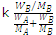For dilute solution,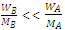and hence,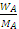.

ΔTƒ = k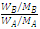= k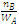MA

If WA is the mass of solvent in kg, then   is equal to molality (m) of the solution

ΔTƒ = kMAm     (? kMA = Kƒ)

ΔTƒ =Kƒm, where Kƒ is called Freezing point depression constant or molal depression constant or cryoscopic constant.

As is clear from the above, depression in freezing point depends upon relative number of moles of solute and solvent but does not depend upon nature of solute, so it is a colligative property.

#### Related Questions in Chemistry

• ##### Q :Reason for medications contain hcl What

What is the reason behind this that some medications contain hcl?

• ##### Q :What are homogenous catalyst? Give few

When a catalyst mixes homogeneously with the reactants and forms a single phase, the catalyst is said to be homogeneous and this type of catalysis is called homogeneous catalysis. Some more examples of homogeneous catalysis are:    SO2

• ##### Q :Molarity of solution Help me to go

Help me to go through this problem. When 7.1gm Na2SO4 (molecular mass 142) dissolves in 100ml H2O , the molarity of the solution is: (a) 2.0 M (b) 1.0 M (c) 0.5 M (d) 0.05 M

• ##### Q :Neutralisation of phosphorous acids

Provide solution of this question. To neutralise completely 20 mL of 0.1 M aqueous solution of phosphorous acid (H3 PO3) the volume of 0.1 M aqueous KOH solution required is: (a) 40 mL (b) 20 mL (c) 10 mL (d) 60 mL

• ##### Q :Problem on solutions The 2N aqueous

The 2N aqueous solution of H2S04 contains: (a) 49 gm of H2S04 per litre of solution (b) 4.9 gm of H2S04 per litre of solution (c) 98 gm of H2S04

• ##### Q :Analytical chemistry 37% weight of HCl

37% weight of HCl and density is 1.1g/ml. find molarity of HCl

• ##### Q :Problem on Molar solution Can someone

Can someone please help me in getting through this problem. 2.0 molar solution is acquired, when 0.5 mole solute is dissolved in: (i) 250 ml solvent (ii) 250 g solvent (iii) 250 ml solution (iv) 1000 ml solvent

• ##### Q :Lab question Explain how dissolving the

Explain how dissolving the Group IV carbonate precipitate with 6M CH3COOH, followed by the addition of extra acetic acid, establishes a buffer with a pH of approximately 5.

• ##### Q :What is electrolysis? Explain with

Passage of a current through a solution can produce an electrolysis reaction.Much additional information on the properties of the ions in an aqueous solution can be obtained from studies of the passage of a direct current (dc) through a cell containing a s

• ##### Q :Theory of three dimensional motion

Partition function; that the translational energy of 1 mol of molecules is 3/2 RT will come as no surprise. But the calculation of this result further illustrates the use of quantized states and the partition function to obtain macroscopic properties. The partition fu